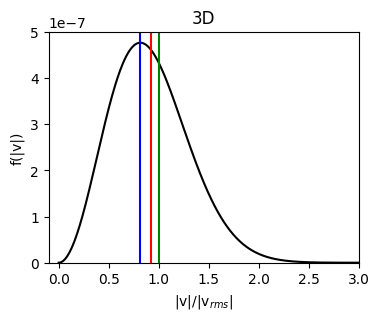# Plasma parameters (`plasmapy.formulary.parameters`)¶

Functions to calculate fundamental plasma parameters.

## Functions¶

 `Alfven_speed`(B, density[, ion, z_mean]) Calculate the Alfvén speed. `Bohm_diffusion`(T_e, B) Return the Bohm diffusion coefficient. `Debye_length`(T_e, n_e) Calculate the characteristic decay length for electric fields, `Debye_number`(T_e, n_e) Return the number of electrons within a sphere with a radius of the Debye length. `gyrofrequency`(B, particle[, signed, Z, to_hz]) Calculate the particle gyrofrequency in units of radians per second. `gyroradius`(B, particle, *[, Vperp, T_i, T]) Return the particle gyroradius. `Hall_parameter`(n, T, B, ion, particle[, ...]) Calculate the `particle` Hall parameter for a plasma. `inertial_length`(n, particle) Calculate a charged particle's inertial length. `ion_sound_speed`(T_e, T_i, ion[, n_e, k, ...]) Return the ion sound speed for an electron-ion plasma. `kappa_thermal_speed`(T, kappa, particle[, method]) Return the most probable speed for a particle within a Kappa distribution. `lower_hybrid_frequency`(B, n_i, ion[, to_hz]) Return the lower hybrid frequency. Calculate the magnetic energy density. Calculate the magnetic pressure. `mass_density`(density, particle[, z_ratio]) Calculate the mass density from a number density. `plasma_frequency`(n, particle[, z_mean, to_hz]) Calculate the particle plasma frequency. Return the thermal pressure for a Maxwellian distribution. `thermal_speed`(T, particle[, method, mass, ndim]) Return the most probable speed for a particle within a Maxwellian distribution. `upper_hybrid_frequency`(B, n_e[, to_hz]) Return the upper hybrid frequency.

## Aliases¶

PlasmaPy provides short-named (alias) versions of the most common plasma functionality. These aliases are only given to functionality where there is a common lexicon in the community, for example `plasma_frequency` has the alias `wp_`. All aliases in PlasmaPy are denoted with a trailing underscore `_`.

 `betaH_`(n, T, B, ion, particle[, ...]) Alias to `Hall_parameter`. `cs_`(T_e, T_i, ion[, n_e, k, gamma_e, ...]) Alias to `ion_sound_speed`. `cwp_`(n, particle) Alias to `inertial_length`. `DB_`(T_e, B) Alias to `Bohm_diffusion`. `lambdaD_`(T_e, n_e) Alias to `Debye_length`. `nD_`(T_e, n_e) Alias to `Debye_number`. `oc_`(B, particle[, signed, Z, to_hz]) Alias to `gyrofrequency`. Alias to `magnetic_pressure`. `pth_`(T, n) Alias to `thermal_pressure`. `rc_`(B, particle, *[, Vperp, T_i, T]) Alias to `gyroradius`. `rho_`(density, particle[, z_ratio]) Alias to `mass_density`. `rhoc_`(B, particle, *[, Vperp, T_i, T]) Alias to `gyroradius`. Alias to `magnetic_energy_density`. `va_`(B, density[, ion, z_mean]) Alias to `Alfven_speed`. `vth_`(T, particle[, method, mass, ndim]) Alias to `thermal_speed`. `vth_kappa_`(T, kappa, particle[, method]) Alias to `kappa_thermal_speed`. `wc_`(B, particle[, signed, Z, to_hz]) Alias to `gyrofrequency`. `wlh_`(B, n_i, ion[, to_hz]) Alias to `lower_hybrid_frequency`. `wp_`(n, particle[, z_mean, to_hz]) Alias to `plasma_frequency`. `wuh_`(B, n_e[, to_hz]) Alias to `upper_hybrid_frequency`.